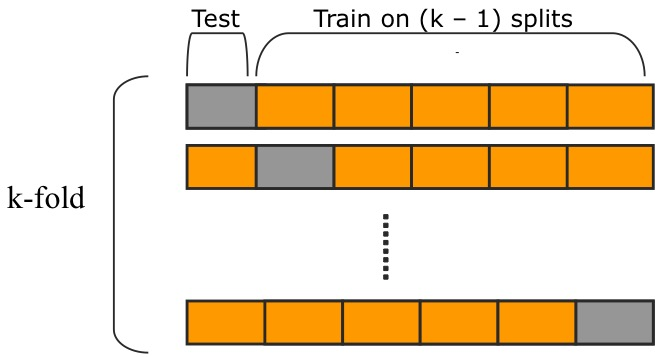# ~ K-fold cross-validation

$K$-fold Cross-validation involves splitting our dataset into $K$ different “folds”. Say, in our example, that we use $K=6$ and divide our dataset into six different folds. First, we use the first fold as our testing set, train on the other five and compute the prediction error of the fitted model. Then, we use the second fold as our testing set and train on the remaining sets, this time also computing the prediction error. Doing this cycle through all $K=6$ folds and averaging the prediction errors gives us our Cross-validation estimate of the prediction error.

An illustration of this from Qinkai’s blog:Denoting the fitted function that excludes the $k$th fold as $\hat f^{-k}(X)$, and defining a function $\kappa:{1,\dots,N}\rightarrow{1,\dots,K}$ that takes in an index of the total dataset and returns the index of the fold containing that datapoint, we can write the Cross-validation estimate of the prediction error as

$$\text{CV}(\hat f) = \frac{1}{N}\sum_{i=1}^NL\left(y_i, \hat f^{-\kappa(i)}(x_i)\right) .$$

With a set of models indexed by the tuning parameter $\alpha$, we see that

$$\text{CV}(\hat f,\alpha) = \frac{1}{N}\sum_{i=1}^NL\left(y_i, \hat f^{-\kappa(i)}(x_i, \alpha)\right)$$

is the curve we need to find the minimum of. The value of $\alpha$ minimizing this curve is often denoted $\hat \alpha$, and we now choose our final model to be $f(x,\hat \alpha)$.